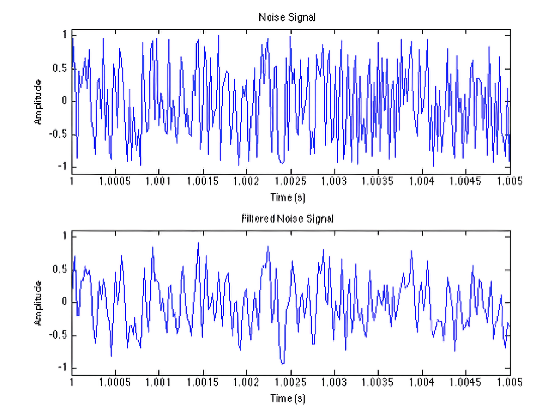﻿ Nathan Paternoster | Digital Audio

# Digital Audio

A study of digital audio and digital signal processing theory. Topics included discrete time sampling, quantization, dithering, analog/digital conversion, signal modulation, digital filtering, and convolution and the Fourier transform. Labs were performed using Matlab.

## Labs

• ### Lab 1 - MATLAB Fundamentals and Sinusoidal Synthesis

Report

In this lab we learned the basics of MATLAB by creating sinusoids, combining multiple sinusoids, and plotting them. We also learned how to import external audio files.

• ### Lab 2 - Sampling, Quantization, and Dither

Report

In this lab we explored the concepts of sampling rates, aliasing, and quantization. We used a function to examine a sine wave quantized to 8, 4, and 2 bits. We also used quantization and random noise to demonstrate the benefits of dither.

• ### Lab 3 - Amplitude and Frequency Modulation

Report

Here we explored the concepts and uses of amplitude modulation (AM) and frequency modulation (FM).

• ### Lab 4 - Basic Filtering

Report

In this lab we began basic filtering operations using the difference equation of a first-order feed-forward filter. We listened to the filtered audio file and plotted the waveform and magnitude spectrum.The effects of a 1st order LPF on a waveform of noise
• ### Lab 5 - Resonant Filter Analysis/Design with MATLAB

Report
Code

In this lab we continued on to more advanced filter design by designing a second-order feedback filter and a second-order resonant filter. The resonant filter was designed to have a cutoff frequency of 2000Hz and a bandwidth of 1000Hz.

• ### Lab 6 - Karplus-Strong String Model

Report
Code
Karplus-Strong Function

This lab explored the Plucked String Karplus-Strong Model - a form of digital synthesis of a plucked string. The aim of this lab was to implement the K-S filter's difference equation and play an impulse and noise signal through it. The resulting sound should resemble a plucked string.

• ### Lab 7 - Convolution and the FFT

Report
Code

This lab focused on convolution and also began discussion on the Discrete Fourier Transform (DFT). We wrote our own two convolution functions. The first function manually performed convolution by summing an input signal with an impulse response of a space. The second function performed convolution by utilizing the Fast Fourier Transform (FFT) and Convolution Theorem - the input signal and impulse response underwent the FFT, were multiplied together, then underwent the inverse FFT. This works because performing the FFT on a signal takes that signal from the time domain to the frequency domain and convolution in the time domain is equivalent to multiplication in the frequency domain (convolution theorem). We compared the two methods by convolving a short snare hit with a room's impulse response and timing how long each method took.

Manual Convolution - Time taken: 306.18s
Convolution using the FFT - Time taken: 0.0163s

• ### Lab 8 - Filtering Using the FFT

Report
Code
Feedback Suppression Filter

In this lab we began using the FFT to filter signals. We created a feedback suppression system that would locate the unwanted sinusoid component (caused by audio feedback) in a signal and then remove it while leaving the rest of the signal intact. For this lab we read in a .wav file and added simulated audio feedback to the file by "injecting" a 4000Hz sinusoid. We then wrote a function that would filter out this 4000Hz frequency from the file by using the FFT.